How to read this lecture...

Code should execute sequentially if run in a Jupyter notebook

# Cass-Koopmans Optimal Growth Model¶

## Contents¶

Coauthor: Brandon Kaplowitz

## Overview¶

This lecture describes a model that Tjalling Koopmans [Koo65] and David Cass [Cas65] used to analyze optimal growth.

The model can be viewed as an extension of the model of Robert Solow described in an earlier lecture but adapted to make the savings rate the outcome of an optimal choice.

(Solow assumed a constant saving rate determined outside the model).

We describe two versions of the model to illustrate what is, in fact, a more general connection between a planned economy and an economy organized as a competitive equilibrium.

The lecture uses important ideas including

• Hicks-Arrow prices named after John R. Hicks and Kenneth Arrow.
• A min-max problem for solving a planning problem.
• A shooting algorithm for solving difference equations subject to initial and terminal conditions.
• A connection between some Lagrange multipliers in the min-max problem and the Hicks-Arrow prices.
• A Big $K$ , little $k$ trick widely used in macroeconomic dynamics.
• An application of a guess and verify method for solving a system of difference equations.
• The intimate connection between the cases for the optimality of two competing visions of good ways to organize an economy, namely:
• socialism in which a central planner commands the allocation of resources, and
• capitalism (also known as a free markets economy) in which competitive equilibrium prices induce individual consumers and producers to choose a socially optimal allocation as an unintended consequence of their completely selfish decisions
• A turnpike property that describes optimal paths for long-but-finite horizon economies.
• A non-stochastic version of a theory of the term structure of interest rates.

In :
from numba import njit
import numpy as np
import matplotlib.pyplot as plt
%matplotlib inline


## The Growth Model¶

Time is discrete and takes values $t = 0, 1 , \ldots, T$.

(We leave open the possibility that $T = + \infty$, but that will require special care in interpreting and using a terminal condition on $K_t$ at $t = T+1$ to be described below).

A single good can either be consumed or invested in physical capital.

The consumption good is not durable and depreciates completely if not consumed immediately.

The capital good is durable but depreciates each period at rate $\delta \in (0,1)$.

We let $C_t$ be a nondurable consumption good at time t.

Let $K_t$ be the stock of physical capital at time t.

Let $\vec{C}$ = $\{C_0,\dots, C_T\}$ and $\vec{K}$ = $\{K_1,\dots,K_{T+1}\}$.

A representative household is endowed with one unit of labor at each $t$ and likes the consumption good at each $t$.

The representative household inelastically supplies a single unit of labor $N_t$ at each $t$, so that $N_t =1 \text{ for all } t \in [0,T]$.

The representative household has preferences over consumption bundles ordered by the utility functional:

$$U(\vec{C}) = \sum_{t=0}^{T} \beta^t \frac{C_t^{1-\gamma}}{1-\gamma} \tag{1}$$

where $\beta \in (0,1)$ is a discount factor and $\gamma >0$ governs the curvature of the one-period utility function.

Note that

$$u(C_t) = \frac{C_t^{1-\gamma}}{1-\gamma} \tag{2}$$

satisfies $u'>0,u''<0$.

$u' > 0$ asserts the consumer prefers more to less.

$u''< 0$ asserts that marginal utility declines with increases in $C_t$.

We assume that $K_0 > 0$ is a given exogenous level of initial capital.

There is an economy-wide production function

$$F(K_t,N_t) = A K_t^{\alpha}N_t^{1-\alpha} \tag{3}$$

with $0 < \alpha<1$, $A > 0$.

A feasible allocation $\vec C, \vec K$ satisfies

$$C_t + K_{t+1} \leq F(K_t,N_t) + (1-\delta) K_t, \quad \text{for all } t \in [0, T] \tag{4}$$

where $\delta \in (0,1)$ is a depreciation rate of capital.

### Planning Problem¶

A planner chooses an allocation $\{\vec{C},\vec{K}\}$ to maximize (1) subject to (4).

Let $\vec{\mu}=\{\mu_0,\dots,\mu_T\}$ be a sequence of nonnegative Lagrange multipliers.

To find an optimal allocation, we form a Lagrangian

$$\mathcal{L}(\vec{C},\vec{K},\vec{\mu}) = \sum_{t=0}^T \beta^t\left\{ u(C_t)+ \mu_t \left(F(K_t,1) + (1-\delta) K_t- C_t - K_{t+1} \right)\right\}$$

and then solve the following min-max problem:

$$\min_{\vec{\mu}} \max_{\vec{C},\vec{K}} \mathcal{L}(\vec{C},\vec{K},\vec{\mu}) \tag{5}$$

#### Useful Properties of Linearly Homogeneous Production Function¶

The following technicalities will help us.

Notice that

$$F(K_t,N_t) = A K_t^\alpha N_t^{1-\alpha} = N_t A\left(\frac{K_t}{N_t}\right)^\alpha$$

Define the output per-capita production function

$$f\left(\frac{K_t}{N_t}\right) = A\left(\frac{K_t}{N_t}\right)^\alpha$$

whose argument is capital per-capita.

Evidently,

$$F(K_t,N_t)=N_t f \left( \frac{K_t}{N_t} \right)$$

Now for some useful calculations.

First

\begin{aligned} \frac{\partial F}{\partial K} &= \frac{\partial N_t f\left( \frac{K_t}{N_t}\right)}{\partial K_t} \\ &= N_t f'\left(\frac{K_t}{N_t}\right)\frac{1}{N_t} \quad \text{(Chain rule)} \\ &= f'\left.\left(\frac{K_t}{N_t}\right)\right|_{N_t=1} \\ &= f'(K_t) \end{aligned} \tag{6}

Also

\begin{aligned} \frac{\partial F}{\partial N} &= \frac{\partial N_t f\left( \frac{K_t}{N_t}\right)}{\partial N_t} \quad \text{(Product rule)} \\ &= f\left(\frac{K_t}{N_t}\right){+} N_t f'\left(\frac{K_t}{N_t}\right) \frac{-K_t}{N_t^2} \quad \text{(Chain rule)} \\ &= f\left(\frac{K_t}{N_t}\right){-}\frac{K_t}{N_t}f'\left.\left(\frac{K_t}{N_t}\right)\right|_{N_t=1} \\ &= f(K_t) - f'(K_t) K_t \end{aligned}

#### Back to Solving the Problem¶

To solve the Lagrangian extremization problem, we compute first derivatives of the Lagrangian and set them equal to 0.

• Note: Our objective function and constraints satisfy conditions that work to assure that required second-order conditions are satisfied at an allocation that satisfies the first-order conditions that we are about to compute.

Here are the first order necessary conditions for extremization (i.e., maximization with respect to $\vec C, \vec K$, minimization with respect to $\vec \mu$):

$$C_t: \qquad u'(C_t)-\mu_t=0 \qquad \text{for all} \quad t= 0,1,\dots,T \tag{7}$$

$$K_t: \qquad \beta \mu_t\left[(1-\delta)+f'(K_t)\right] - \mu_{t-1}=0 \qquad \text{for all } \quad t=1,2,\dots,T \tag{8}$$

$$\mu_t:\qquad F(K_t,1)+ (1-\delta) K_t - C_t - K_{t+1}=0 \qquad \text{for all } \quad t=0,1,\dots,T \tag{9}$$

$$K_{T+1}: \qquad -\mu_T \leq 0, \ \leq 0 \text{ if } K_{T+1}=0; \ =0 \text{ if } K_{T+1}>0 \tag{10}$$

Note that in (8) we plugged in for $\frac{\partial F}{\partial K}$ using our formula (6) above.

Because $N_t = 1$ for $t = 1, \ldots, T$, need not differentiate with respect to those arguments.

Note that (9) comes from the occurrence of $K_t$ in both the period $t$ and period $t-1$ feasibility constraints.

(10) comes from differentiating with respect to $K_{T+1}$ in the last period and applying the following condition called a Karush-Kuhn-Tucker condition (KKT):

$$\mu_T K_{T+1}=0 \tag{11}$$

Combining (7) and (8) gives

$$u'\left(C_t\right)\left[(1-\delta)+f'\left(K_t\right)\right]-u'\left(C_{t-1}\right)=0 \quad \text{ for all } t=1,2,\dots, T+1$$

Rewriting gives

$$u'\left(C_{t+1}\right)\left[(1-\delta)+f'\left(K_{t+1}\right)\right]= u'\left(C_{t}\right) \quad \text{ for all } t=0,1,\dots, T \tag{12}$$

Taking the inverse of the utility function on both sides of the above equation gives

$$C_{t+1} =u'^{-1}\left(\left(\frac{\beta}{u'(C_t)}[f'(K_{t+1}) +(1-\delta)]\right)^{-1}\right)$$

or using our utility function (2)

\begin{aligned} C_{t+1} =\left(\beta C_t^{\gamma}[f'(K_{t+1}) + (1-\delta)]\right)^{1/\gamma} \notag\\= C_t\left(\beta [f'(K_{t+1}) + (1-\delta)]\right)^{1/\gamma} \end{aligned}

The above first-order condition for consumption is called an Euler equation.

It tells us how consumption in adjacent periods are optimally related to each other and to capital next period.

We now use some of the equations above to calculate some variables and functions that we’ll soon use to solve the planning problem with Python.

In :
@njit
def u(c, γ):
'''
Utility function
ASIDE: If you have a utility function that is hard to solve by hand
you can use automatic or symbolic  differentiation
'''
if γ == 1:
## If γ = 1 we can show via L'hopital's Rule that the utility becomes log
return np.log(c)
else:
return c**(1 - γ) / (1 - γ)

@njit
def u_prime(c, γ):
'''Derivative of utility'''
if γ == 1:
return 1 / c
else:
return c**(-γ)

@njit
def u_prime_inv(c, γ):
'''Inverse utility'''
if γ == 1:
return c
else:
return c**(-1 / γ)

@njit
def f(A, k, α):
'''Production function'''
return A * k**α

@njit
def f_prime(A, k, α):
'''Derivative of production function'''
return α * A * k**(α - 1)

@njit
def f_prime_inv(A, k, α):
return (k / (A * α))**(1 / (α - 1))


### Shooting Method¶

We shall use a shooting method to compute an optimal allocation $\vec C, \vec K$ and an associated Lagrange multiplier sequence $\vec \mu$.

The first-order necessary conditions for the planning problem, namely, equations (7), (8), and (9), form a system of difference equations with two boundary conditions:

• $K_0$ is a given initial condition for capital
• $K_{T+1} =0$ is a terminal condition for capital that we deduced from the first-order necessary condition for $K_{T+1}$ the KKT condition (11)

We have no initial condition for the Lagrange multiplier $\mu_0$.

If we did, solving for the allocation would be simple:

• Given $\mu_0$ and $k_0$, we could compute $c_0$ from equation (7) and then $k_1$ from equation (9) and $\mu_1$ from equation (8).
• We could then iterate on to compute the remaining elements of $\vec C, \vec K, \vec \mu$.

But we don’t have an initial condition for $\mu_0$, so this won’t work.

But a simple modification called the shooting algorithm will work.

The shooting algorithm is an instance of a guess and verify algorithm.

It proceeds as follows:

• Guess a value for the initial Lagrange multiplier $\mu_0$.
• Apply the simple algorithm described above.
• Compute the implied value of $k_{T+1}$ and check whether it equals zero.
• If the implied $K_{T+1} =0$, we have solved the problem.
• If $K_{T+1} > 0$, lower $\mu_0$ and try again.
• If $K_{T+1} < 0$, raise $\mu_0$ and try again.

The following Python code implements the shooting algorithm for the planning problem.

We make a slight modification starting with a guess of $c_0$ but since $c_0$ is a function of $\mu_0$ there is no difference to the procedure above.

We’ll apply it with an initial guess that will turn out not to be perfect, as we’ll soon see.

In :
# Parameters
γ = 2
δ = 0.02
β = 0.95
α = 0.33
A = 1

# Initial guesses
T = 10
c = np.zeros(T+1)  # T periods of consumption initialized to 0
k = np.zeros(T+2)  # T periods of capital initialized to 0 (T+2 to include t+1 variable as well)
k = 0.3  # Initial k
c = 0.2  # Guess of c_0

@njit
def shooting_method(c, # Initial consumption
k,   # Initial capital
γ,   # Coefficient of relative risk aversion
δ,   # Depreciation rate on capital# Depreciation rate
β,   # Discount factor
α,   # Return to capital per capita
A):  # Technology

T = len(c) - 1

for t in range(T):
k[t+1] = f(A=A, k=k[t], α=α) + (1 - δ) * k[t] - c[t]  # Equation 1 with inequality
if k[t+1] < 0:   # Ensure nonnegativity
k[t+1] = 0

# Equation 2: We keep in the general form to show how we would
# solve if we didn't want to do any simplification

if β * (f_prime(A=A, k=k[t+1], α=α) + (1 - δ)) == np.inf:
# This only occurs if k[t+1] is 0, in which case, we won't
# produce anything next period, so consumption will have to be 0
c[t+1] = 0
else:
c[t+1] = u_prime_inv(u_prime(c=c[t], γ=γ) / (β * (f_prime(A=A, k=k[t+1], α=α) + (1 - δ))), γ=γ)

# Terminal condition calculation
k[T+1] = f(A=A, k=k[T], α=α) + (1 - δ) * k[T] - c[T]

return c, k

paths = shooting_method(c, k, γ, δ, β, α, A)

fig, axes = plt.subplots(1, 2, figsize=(10, 4))
colors = ['blue', 'red']
titles = ['Consumption', 'Capital']
ylabels = ['$c_t$', '$k_t$']

for path, color, title, y, ax in zip(paths, colors, titles, ylabels, axes):
ax.plot(path, c=color, alpha=0.7)
ax.set(title=title, ylabel=y, xlabel='t')

ax.scatter(T+1, 0, s=80)
ax.axvline(T+1, color='k', ls='--', lw=1)

plt.tight_layout()
plt.show()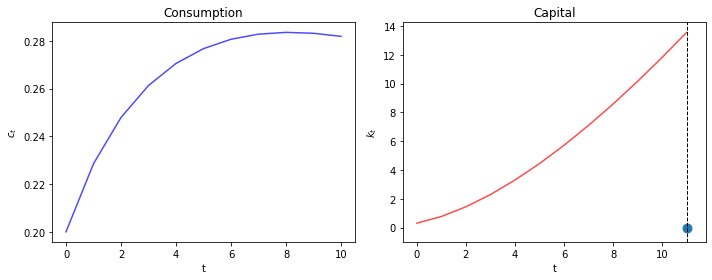Evidently, our initial guess for $\mu_0$ is too high and makes initial consumption is too low.

We know this because we miss our $K_{T+1}=0$ target on the high side.

Now we automate things with a search-for-a-good $\mu_0$ algorithm that stops when we hit the target $K_{t+1} = 0$.

The search procedure is to use a bisection method.

Here is how we apply the bisection method.

We take an initial guess for $C_0$ (we can eliminate $\mu_0$ because $C_0$ is an exact function of $\mu_0$).

We know that the lowest $C_0$ can ever be is $0$ and the largest it can be is initial output $f(K_0)$.

We take a $C_0$ guess and shoot forward to $T+1$.

If the $K_{T+1}>0$, let it be our new lower bound on $C_0$.

If $K_{T+1}<0$, let it be our new upper bound.

Make a new guess for $C_0$ exactly halfway between our new upper and lower bounds.

Shoot forward again and iterate the procedure.

When $K_{T+1}$ gets close enough to 0 (within some error tolerance bounds), stop and declare victory.

In :
@njit
def bisection_method(c,
k,
γ,              # Coefficient of relative risk aversion
δ,              # Depreciation rate on capital# Depreciation rate
β,              # Discount factor
α,              # Return to capital per capita
A,              # Technology
tol=1e-4,
max_iter=1e4,
terminal=0):    # Value we are shooting towards

T = len(c) - 1
i = 1                            # Initial iteration
c_high = f(k=k, α=α, A=A)     # Initial high value of c
c_low = 0                        # Initial low value of c

path_c, path_k = shooting_method(c, k, γ, δ, β, α, A)

while (np.abs((path_k[T+1] - terminal)) > tol or path_k[T] == terminal) and i < max_iter:

if path_k[T+1] - terminal > tol:
# If assets are too high the c we chose is now a lower bound on possible values of c
c_low = c
elif path_k[T+1] - terminal < -tol:
# If assets fell too quickly, the c we chose is now an upper bound on possible values of c
c_high=c
elif path_k[T] == terminal:
# If assets fell  too quickly, the c we chose is now an upper bound on possible values of c
c_high=c

c = (c_high + c_low) / 2  # This is the bisection part
path_c, path_k = shooting_method(c, k, γ, δ, β, α, A)
i += 1

if np.abs(path_k[T+1] - terminal) < tol and path_k[T] != terminal:
print('Converged successfully on iteration', i-1)
else:
print('Failed to converge and hit maximum iteration')

μ = u_prime(c=path_c, γ=γ)
return path_c, path_k, μ


Now we can plot

In :
T = 10
c = np.zeros(T+1) # T periods of consumption initialized to 0
k = np.zeros(T+2) # T periods of capital initialized to 0. T+2 to include t+1 variable as well.

k = 0.3 # initial k
c = 0.3 # our guess of c_0

paths = bisection_method(c, k, γ, δ, β, α, A)

def plot_paths(paths, axes=None, ss=None):

T = len(paths)

if axes is None:
fix, axes = plt.subplots(1, 3, figsize=(13, 3))

ylabels = ['$c_t$', '$k_t$', '$\mu_t$']
titles = ['Consumption', 'Capital', 'Lagrange Multiplier']

for path, y, title, ax in zip(paths, ylabels, titles, axes):
ax.plot(path)
ax.set(ylabel=y, title=title, xlabel='t')

# Plot steady state value of capital
if ss is not None:
axes.axhline(ss, c='k', ls='--', lw=1)

axes.axvline(T, c='k', ls='--', lw=1)
axes.scatter(T, paths[-1], s=80)
plt.tight_layout()

plot_paths(paths)

Converged successfully on iteration 18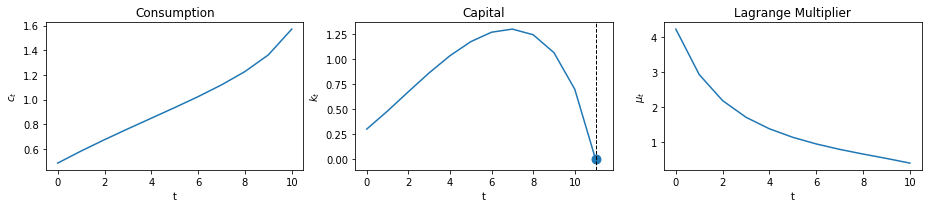### Setting Intial Capital to the Steady State¶

If $T \rightarrow +\infty$, the optimal allocation converges to steady state values of $C_t$ and $K_t$.

It is instructive to compute these and then to set $K_0$ equal to its steady state value.

In a steady state $K_{t+1} = K_t=\bar{K}$ for all very large $t$ the feasibility constraint (4) is

$$f(\bar{K})-\delta \bar{K} = \bar{C} \tag{13}$$

Substituting $K_t = \bar K$ and $C_t=\bar C$ for all $t$ into (12) gives

$$1=\beta \frac{u'(\bar{C})}{u'(\bar{C})}[f'(\bar{K})+(1-\delta)]$$

Defining $\beta = \frac{1}{1+\rho}$, and cancelling gives

$$1+\rho = 1[f'(\bar{K}) + (1-\delta)]$$

Simplifying gives

$$f'(\bar{K}) = \rho +\delta$$

and

$$\bar{K} = f'^{-1}(\rho+\delta)$$

Using our production function (3) gives

$$\alpha \bar{K}^{\alpha-1} = \rho + \delta$$

Finally, using $\alpha= .33$, $\rho = 1/\beta-1 =1/(19/20)-1 = 20/19-19/19 = 1/19$, $\delta = 1/50$, we get

$$\bar{K} = \left(\frac{\frac{33}{100}}{\frac{1}{50}+\frac{1}{19}}\right)^{\frac{67}{100}} \approx 9.57583$$

Let’s verify this with Python and then use this steady state $\bar K$ as our initial capital stock $K_0$.

In :
ρ = 1 / β - 1
k_ss = f_prime_inv(k=ρ+δ, A=A, α=α)

print(f'steady state for capital is: {k_ss}')

steady state for capital is: 9.57583816331462


Now we plot

In :
T = 150
c = np.zeros(T+1)
k = np.zeros(T+2)
c = 0.3
k = k_ss  # Start at steady state
paths = bisection_method(c, k, γ, δ, β, α, A)

plot_paths(paths, ss=k_ss)

Converged successfully on iteration 39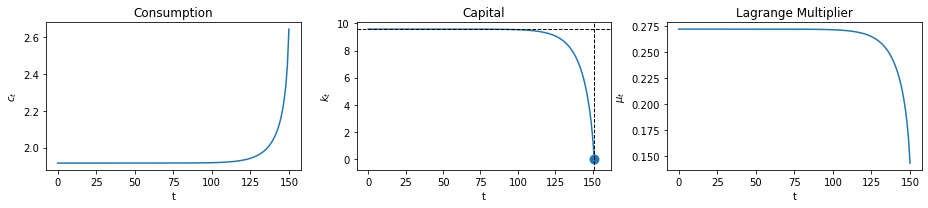Evidently, in this economy with a large value of $T$, $K_t$ stays near its initial value at the until the end of time approaches closely.

Evidently, the planner likes the steady state capital stock and wants to stay near there for a long time.

Let’s see what happens when we push the initial $K_0$ below $\bar K$.

In :
k_init = k_ss / 3   # Below our steady state
T = 150
c = np.zeros(T+1)
k = np.zeros(T+2)
c = 0.3
k = k_init
paths = bisection_method(c, k, γ, δ, β, α, A)

plot_paths(paths, ss=k_ss)

Converged successfully on iteration 39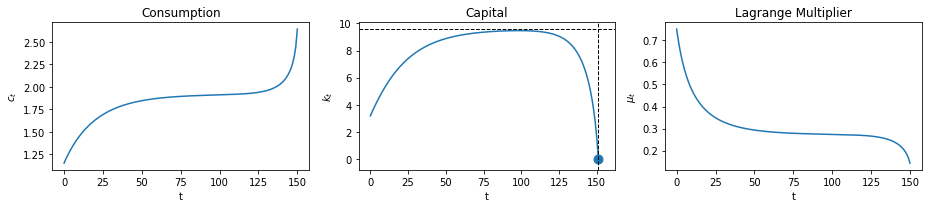Notice how the planner pushes capital toward the steady state, stays near there for a while, then pushes $K_t$ toward the terminal value $K_{T+1} =0$ as $t$ gets close to $T$.

The following graphs compare outcomes as we vary $T$.

In :
T_list = (150, 75, 50, 25)

fix, axes = plt.subplots(1, 3, figsize=(13, 3))

for T in T_list:
c = np.zeros(T+1)
k = np.zeros(T+2)
c = 0.3
k = k_init
paths = bisection_method(c, k, γ, δ, β, α, A)
plot_paths(paths, ss=k_ss, axes=axes)

Converged successfully on iteration 39
Converged successfully on iteration 26
Converged successfully on iteration 25
Converged successfully on iteration 22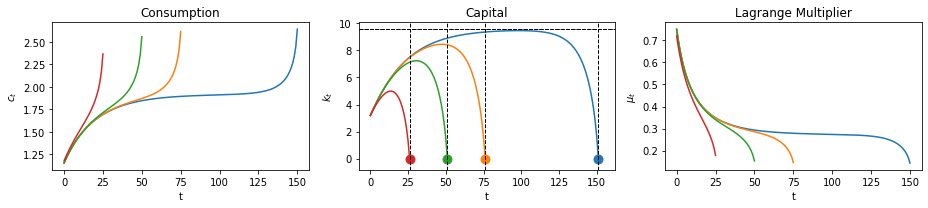The following calculation shows that when we set $T$ very large the planner makes the capital stock spend most of its time close to its steady state value.

In :
T_list = (250, 150, 50, 25)

fix, axes = plt.subplots(1, 3, figsize=(13, 3))

for T in T_list:
c = np.zeros(T+1)
k = np.zeros(T+2)
c = 0.3
k = k_init
paths = bisection_method(c, k, γ, δ, β, α, A)
plot_paths(paths, ss=k_ss, axes=axes)

Failed to converge and hit maximum iteration
Converged successfully on iteration 39
Converged successfully on iteration 25
Converged successfully on iteration 22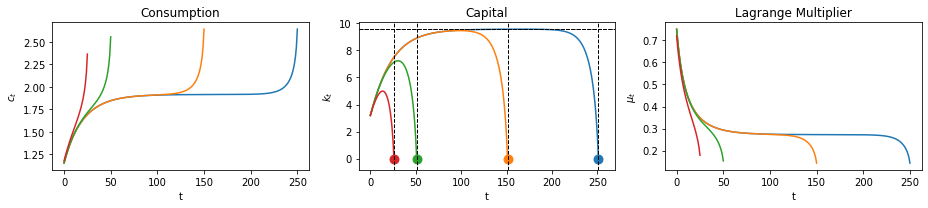The different colors in the above graphs are tied to outcomes with different horizons $T$.

Notice that as the horizon increases, the planner puts $K_t$ closer to the steady state value $\bar K$ for longer.

This pattern reflects a turnpike property of the steady state.

A rule of thumb for the planner is

• for whatever $K_0$ you start with, push $K_t$ toward the steady state and stay there for as long as you can

In loose language: head for the turnpike and stay near it for as long as you can.

As we drive $T$ toward $+\infty$, the planner keeps $K_t$ very close to its steady state for all dates after some transition toward the steady state.

The planner makes the saving rate $\frac{f(K_t) - C_t}{f(K_t)}$ vary over time.

Let’s calculate it

In :
@njit
def S(K):
'''Aggregate savings'''
T = len(K) - 2
S = np.zeros(T+1)
for t in range(T+1):
S[t] = K[t+1] - (1 - δ) * K[t]
return S

@njit
def s(K):
'''Savings rate'''
T = len(K) - 2
Y = f(A, K, α)
Y = Y[0:T+1]
s = S(K) / Y
return s

def plot_savings(paths, c_ss=None, k_ss=None, s_ss=None, axes=None):

T = len(paths)
k_star = paths
savings_path = s(k_star)
new_paths = (paths, paths, savings_path)

if axes is None:
fix, axes = plt.subplots(1, 3, figsize=(13, 3))

ylabels = ['$c_t$', '$k_t$', '$s_t$']
titles = ['Consumption', 'Capital', 'Savings Rate']

for path, y, title, ax in zip(new_paths, ylabels, titles, axes):
ax.plot(path)
ax.set(ylabel=y, title=title, xlabel='t')

# Plot steady state value of consumption
if c_ss is not None:
axes.axhline(c_ss, c='k', ls='--', lw=1)

# Plot steady state value of capital
if k_ss is not None:
axes.axhline(k_ss, c='k', ls='--', lw=1)

# Plot steady state value of savings
if s_ss is not None:
axes.axhline(s_ss, c='k', ls='--', lw=1)

axes.axvline(T, c='k', ls='--', lw=1)
axes.scatter(T, k_star[-1], s=80)
plt.tight_layout()

T_list = (250, 150, 75, 50)

fix, axes = plt.subplots(1, 3, figsize=(13, 3))

for T in T_list:
c = np.zeros(T+1)
k = np.zeros(T+2)
c = 0.3
k = k_init
paths = bisection_method(c, k, γ, δ, β, α, A)
plot_savings(paths, k_ss=k_ss, axes=axes)

Failed to converge and hit maximum iteration
Converged successfully on iteration 39
Converged successfully on iteration 26
Converged successfully on iteration 25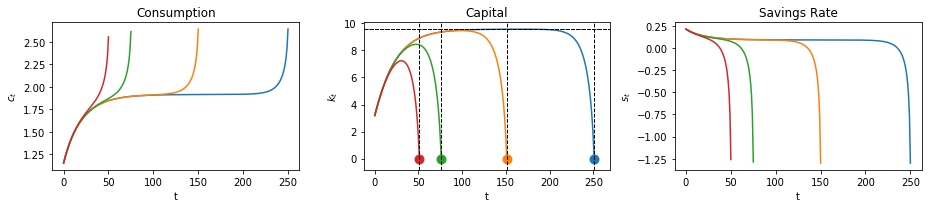### The Limiting Economy¶

We now consider an economy in which $T = +\infty$.

The appropriate thing to do is to replace terminal condition (10) with

$$\lim_{T \rightarrow +\infty} \beta^T u'(C_T) K_{T+1} = 0$$

which is sometimes called a transversality condition.

This condition will be satisfied by a path that converges to an optimal steady state.

We can approximate the optimal path from an arbitrary initial $K_0$ and shooting towards the optimal steady state $K$ at a large but finite $T+1$.

In the following code, we do this for a large $T$; we shoot towards the steady state and plot consumption, capital and the savings rate.

We know that in the steady state that the saving rate must be fixed and that $\bar s= \frac{f(\bar K)-\bar C}{f(\bar K)}$.

From (13) the steady state saving rate equals

$$\bar s =\frac{ \delta \bar{K}}{f(\bar K)}$$

The steady state savings level $\bar S = \bar s f(\bar K)$ is the amount required to offset capital depreciation each period.

We first study optimal capital paths that start below the steady state

In :
T = 130

S_ss = δ * k_ss
c_ss = f(A, k_ss, α) - S_ss
s_ss = S_ss / f(A, k_ss, α)

c = np.zeros(T+1)
k = np.zeros(T+2)
c = 0.3
k = k_ss / 3         # Start below steady state
paths = bisection_method(c, k, γ, δ, β, α, A, terminal=k_ss)
plot_savings(paths, k_ss=k_ss, s_ss=s_ss, c_ss=c_ss)

Converged successfully on iteration 35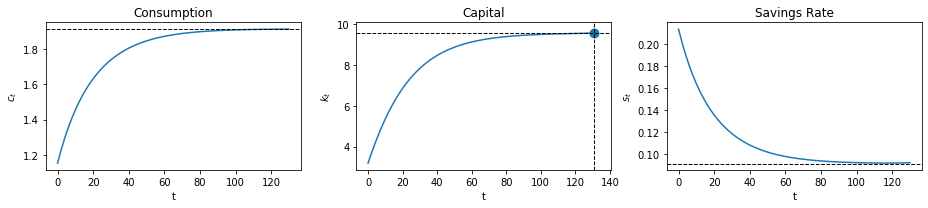Since $K_0<\bar K$, $f'(K_0)>\rho +\delta$.

The planner chooses a positive saving rate above the steady state level offsetting depreciation that enables us to increase our capital stock.

Note, $f''(K)<0$, so as $K$ rises, $f'(K)$ declines.

The planner slowly lowers the savings rate until reaching a steady state where $f'(K)=\rho +\delta$.

### Exercise¶

• Plot the optimal consumption, capital, and savings paths when the initial capital level begins at 1.5 times the steady state level as we shoot towards the steady state at $T=130$.
• Why does the savings rate respond like it does?

### Solution¶

In :
T = 130

c = np.zeros(T+1)
k = np.zeros(T+2)
c = 0.3
k = k_ss * 1.5   # Start above steady state
paths = bisection_method(c, k, γ, δ, β, α, A, terminal=k_ss)
plot_savings(paths, k_ss=k_ss, s_ss=s_ss, c_ss=c_ss)

Converged successfully on iteration 31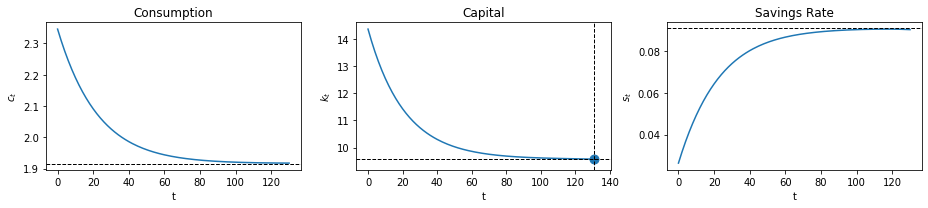## Competitive Equilibrium¶

Next, we study a decentralized version of an economy with the same technology and preference structure as our planned economy.

But now there is no planner.

Market prices adjust to reconcile distinct decisions that are made separately by a representative household and a representative firm.

The technology for producing goods and accumulating capital via physical investment remains as in our planned economy.

There is a representative consumer who has the same preferences over consumption plans as did the consumer in the planned economy.

Instead of being told what to consume and save by a planner, the household chooses for itself subject to a budget constraint

• At each time $t$, the household receives wages and rentals of capital from a firm – these comprise its income at time $t$.
• The consumer decides how much income to allocate to consumption or to savings.
• The household can save either by acquiring additional physical capital (it trades one for one with time $t$ consumption) or by acquiring claims on consumption at dates other than $t$.
• A utility-maximizing household owns all physical capital and labor and rents them to the firm.
• The household consumes, supplies labor, and invests in physical capital.
• A profit-maximizing representative firm operates the production technology.
• The firm rents labor and capital each period from the representative household and sells its output each period to the household.
• The representative household and the representative firm are both price takers:
• they (correctly) believe that prices are not affected by their choices

Note: We are free to think of there being a large number $M$ of identical representative consumers and $M$ identical representative firms.

### Firm Problem¶

At time $t$ the representative firm hires labor $\tilde n_t$ and capital $\tilde k_t$.

The firm’s profits at time $t$ are

$$F(\tilde k_t, \tilde n_t)-w_t \tilde n_t -\eta_t \tilde k_t$$

where $w_t$ is a wage rate at $t$ and $\eta_t$ is the rental rate on capital at $t$.

As in the planned economy model

$$F(\tilde k_t, \tilde n_t) = A \tilde k_t^\alpha \tilde n_t^{1-\alpha}$$

#### Zero Profit Conditions¶

Zero-profits condition for capital and labor are

$$F_k(\tilde k_t, \tilde n_t) =\eta_t$$

and

$$F_n(\tilde k_t, \tilde n_t) =w_t \tag{14}$$

These conditions emerge from a no-arbitrage requirement.

To describe this line of reasoning, we begin by applying a theorem of Euler about linearly homogenous functions.

The theorem applies to the Cobb-Douglas production function because it assumed displays constant returns to scale:

$$\alpha F(\tilde k_t, \tilde n_t) = F(\alpha \tilde k_t, \alpha \tilde n_t)$$

for $\alpha \in (0,1)$.

Taking the partial derivative $\frac{\partial F }{\partial \alpha}$ on both sides of the above equation gives

$$F(\tilde k_t,\tilde n_t) =_\text{chain rule} \frac{\partial F}{\partial \tilde k_t} \tilde k_t + \frac{\partial F}{\partial \tilde n_t} \tilde n_t$$

Rewrite the firm’s profits as

$$\frac{\partial F}{\partial \tilde k_t} \tilde k_t + \frac{\partial F}{\partial \tilde n_t} \tilde n_t-w_t \tilde n_t -\eta_t k_t$$

or

$$\left(\frac{\partial F}{\partial \tilde k_t}-\eta_t\right) \tilde k_t + \left(\frac{\partial F}{\partial \tilde n_t}-w_t\right) \tilde n_t$$

Because $F$ is homogeneous of degree $1$, it follows that $\frac{\partial F}{\partial \tilde k_t}$ and $\frac{\partial F}{\partial \tilde n_t}$ are homogeneous of degree $0$ and therefore fixed with respect to $\tilde k_t$ and $\tilde n_t$.

If $\frac{\partial F}{\partial \tilde k_t}> \eta_t$, then the firm makes positive profits on each additional unit of $\tilde k_t$, so it will want to make $\tilde k_t$ arbitrarily large.

But setting $\tilde k_t = + \infty$ is not physically feasible, so presumably equilibrium prices will assume values that present the firm with no such arbitrage opportunity.

A related argument applies if $\frac{\partial F}{\partial \tilde n_t}> w_t$.

If $\frac{\partial \tilde k_t}{\partial \tilde k_t}< \eta_t$, the firm will set $\tilde k_t$ to zero.

Again, equilibrium prices won’t incentive the firm to do that.

And so on…

It is convenient to define $\vec w_t =\{w_0, \dots,w_T\}$and $\vec \eta_t = \{\eta_0, \dots, \eta_T\}$.

### Household Problem¶

A representative household lives at $t=0,1,\dots, T$.

At $t$, the household rents $1$ unit of labor and $k_t$ units of capital to a firm and receives income

$$w_t 1+ \eta_t k_t$$

At $t$ the household allocates its income to the following purchases

$$\left(c_t + (k_{t+1} -(1-\delta)k_t)\right)$$

Here $\left(k_{t+1} -(1-\delta)k_t\right)$ is the household’s net investment in physical capital and $\delta \in (0,1)$ is again a depreciation rate of capital.

In period $t$ is free to purchase more goods to be consumed and invested in physical capital than its income from supplying capital and labor to the firm, provided that in some other periods its income exceeds its purchases.

A household’s net excess demand for time $t$ consumption goods is the gap

$$e_t \equiv \left(c_t + (k_{t+1} -(1-\delta)k_t)\right)-(w_t 1 + \eta_t k_t)$$

Let $\vec c = \{c_0,\dots,c_T\}$ and let $\vec k = \{k_1,\dots,k_{T+1}\}$.

$k_0$ is given to the household.

### Market Structure for Intertemporal Trades¶

There is a single grand competitive market in which a representative household can trade date $0$ goods for goods at all other dates $t=1, 2, \ldots, T$.

What matters are not bilateral trades of the good at one date $t$ for the good at another date $\tilde t \neq t$.

Instead, think of there being multilateral and multitemporal trades in which bundles of goods at some dates can be traded for bundles of goods at some other dates.

There exist complete markets in such bundles with associated market prices.

### Market Prices¶

Let $q^0_t$ be the price of a good at date $t$ relative to a good at date $0$.

$\{q^0_t\}_{t=0}^T$ is a vector of Hicks-Arrow prices, named after the 1972 joint economics Nobel prize winners who used such prices in some of their important work.

Evidently,

$$q^0_t=\frac{\text{number of time 0 goods}}{\text{number of time t goods}}$$

Because $q^0_t$ is a relative price, the units in terms of which prices are quoted are arbitrary – we can normalize them without substantial consequence.

If we use the price vector $\{q^0_t\}_{t=0}^T$ to evaluate a stream of excess demands $\{e_t\}_{t=0}^T$ we compute the present value of $\{e_t\}_{t=0}^T$ to be $\sum_{t=0}^T q^0_t e_t$.

That the market is multitemporal is reflected in the situation that the household faces a single budget constraint.

It states that the present value of the household’s net excess demands must be zero:

$$\sum_{t=0}^T q^0_t e_t \leq 0$$

or

$$\sum_{t=0}^T q^0_t \left(c_t + (k_{t+1} -(1-\delta)k_t)-(w_t 1 + \eta_t k_t) \right) \leq 0$$

### Household Problem¶

The household faces the constrained optimization problem:

\begin{aligned}& \max_{\vec c, \vec k} \sum_{t=0}^T \beta^t u(c_t) \\ \text{subject to} \ \ & \sum_{t=0}^T q_t^0\left(c_t +\left(k_{t+1}-(1-\delta) k_t\right) -w_t -\eta_t k_t\right) \leq 0 \notag \end{aligned}

### Definitions¶

• A price system is a sequence $\{q_t^0,\eta_t,w_t\}_{t=0}^T= \{\vec q, \vec \eta, \vec w\}$.
• An allocation is a sequence $\{c_t,k_{t+1},n_t=1\}_{t=0}^T = \{\vec c, \vec k, \vec n =1\}$.
• A competitive equilibrium is a price system and an allocation for which
• Given the price system, the allocation solves the household’s problem.
• Given the price system, the allocation solves the firm’s problem.

### Computing a Competitive Equilibrium¶

We shall compute a competitive equilibrium using a guess and verify approach.

• We shall guess equilibrium price sequences $\{\vec q, \vec \eta, \vec w\}$.
• We shall then verify that at those prices, the household and the firm choose the same allocation.

#### Guess for Price System¶

We have computed an allocation $\{\vec C, \vec K, \vec 1\}$ that solves the planning problem.

We use that allocation to construct our guess for the equilibrium price system.

In particular, we guess that for $t=0,\dots,T$:

$$\lambda q_t^0 = \beta^t u'(K_t) =\beta^t \mu_t \tag{15}$$

$$w_t = f(K_t) -K_t f'(K_t) \tag{16}$$

$$\eta_t = f'(K_t) \tag{17}$$

At these prices, let the capital chosen by the household be

$$k^*_t(\vec q, \vec w, \vec \eta) , \quad t \geq 0 \tag{18}$$

and let the allocation chosen by the firm be

$$\tilde k^*_t(\vec q, \vec w, \vec \eta), \quad t \geq 0$$

and so on.

If our guess for the equilibrium price system is correct, then it must occur that

$$k_t^* = \tilde k_t^* \tag{19}$$

$$1 = \tilde n_t^* \tag{20}$$

$$c_t^* + k_{t+1}^* - (1-\delta) k_t^* = F(\tilde k_t^*, \tilde n_t^*)$$

We shall verify that for $t=0,\dots,T$ the allocations chosen by the household and the firm both equal the allocation that solves the planning problem:

$$k^*_t = \tilde k^*_t=K_t, \tilde n_t=1, c^*_t=C_t \tag{21}$$

### Verification Procedure¶

Our approach is to stare at first-order necessary conditions for the optimization problems of the household and the firm.

At the price system we have guessed, both sets of first-order conditions are satisfied at the allocation that solves the planning problem.

### Household’s Lagrangian¶

To solve the household’s problem, we formulate the appropriate Lagrangian and pose the min-max problem:

$$\min_{\lambda} \max_{\vec{c},\vec{k}}\mathcal{L}(\vec{c},\vec{k},\lambda)= \sum_{t=0}^T \beta^t u(c_t)+ \lambda \left(\sum_{t=0}^T q_t^0\left(\left((1-\delta) k_t -w_t\right) +\eta_t k_t -c_t - k_{t+1}\right)\right)$$

First-order conditions are

$$c_t: \quad \beta^t u'(c_t)-\lambda q_t^0=0 \quad t=0,1,\dots,T \tag{22}$$

$$k_t: \quad -\lambda q_t^0 \left[(1-\delta)+\eta_t \right]+\lambda q^0_{t-1}=0 \quad t=1,2,\dots,T+1 \tag{23}$$

$$\lambda: \quad \left(\sum_{t=0}^T q_t^0\left(c_t + \left(k_{t+1}-(1-\delta) k_t\right) -w_t -\eta_t k_t\right)\right) \leq 0 \tag{24}$$

$$k_{T+1}: \quad -\lambda q_0^{T+1} \leq 0, \ \leq 0 \text{ if } k_{T+1}=0; \ =0 \text{ if } k_{T+1}>0 \tag{25}$$

Now we plug in for our guesses of prices and derive all the FONC of the planner problem (7)-(10):

Combining (22) and (15), we get:

$$u'(C_t) = \mu_t$$

which is (7).

Combining (23), (15), and (17) we get:

$$-\lambda \beta^t \mu_t\left[(1-\delta) +f'(K_t)\right] +\lambda \beta^{t-1}\mu_{t-1}=0 \tag{26}$$

Rewriting (26) by dividing by $\lambda$ on both sides (which is nonzero due to u’>0) we get:

$$\beta^t \mu_t [(1-\delta+f'(K_t)] = \beta^{t-1} \mu_{t-1}$$

or

$$\beta \mu_t [(1-\delta+f'(K_t)] = \mu_{t-1}$$

which is (8).

Combining (24), (15), (16) and (17) after multiplying both sides of (24) by $\lambda$, we get:

$$\sum_{t=0}^T \beta^t \mu_{t} \left(C_t+ (K_{t+1} -(1-\delta)K_t)-f(K_t)+K_t f'(K_t)-f'(K_t)K_t\right) \leq 0$$

Cancelling,

$$\sum_{t=0}^T \beta^t \mu_{t} \left(C_t +K_{t+1} -(1-\delta)K_t - F(K_t,1)\right) \leq 0$$

Since $\beta^t$ and $\mu_t$ are always positive here, (excepting perhaps the T+1 period) we get:

$$C_t+K_{t+1}-(1-\delta)K_t -F(K_t,1)=0 \quad \text{ for all }t \text{ in } 0,\dots,T$$

which is (9).

Combining (25) and (15), we get:

$$- \beta^{T+1} \mu_{T+1} \leq 0$$

Dividing both sides by $\beta^{T+1}$ which will be strictly positive here, we get:

$$-\mu_{T+1} \leq 0$$

which is the (10) of our planning problem.

Thus, at our guess of the equilibrium price system, the allocation that solves the planning problem also solves the problem faced by a representative household living in a competitive equilibrium.

We now consider the problem faced by a firm in a competitive equilibrium:

If we plug in (21) into (14) for all t, we get

$$\frac{\partial F(K_t, 1)}{\partial K_t} = f'(K_t) = \eta_t$$

which is (17).

If we now plug (21) into (14) for all t, we get:

$$\frac{\partial F(\tilde K_t, 1)}{\partial \tilde L} = f(K_t)-f'(K_t)K_t=w_t$$

which is exactly (18).

Thus, at our guess of the equilibrium price system, the allocation that solves the planning problem also solves the problem faced by a firm within a competitive equilibrium.

By (19) and (20) this allocation is identical to the one that solves the consumer’s problem.

Note: Because budget sets are affected only by relative prices, $\{q_0^t\}$ is determined only up to multiplication by a positive constant.

Normalization: We are free to choose a $\{q_0^t\}$ that makes $\lambda=1$, thereby making $q_0^t$ be measured in units of the marginal utility of time $0$ goods.

We will also plot q, w and $\eta$ below to show the prices that induce the same aggregate movements we saw earlier in the planning problem.

In :
@njit
def q_func(β, c, γ):
# Here we choose numeraire to be u'(c_0) -- this is q^(t_0)_t
T = len(c) - 2
q = np.zeros(T+1)
q = 1
for t in range(1, T+2):
q[t] = β**t * u_prime(c[t], γ)
return q

@njit
def w_func(A, k, α):
w = f(A, k, α) - k * f_prime(A, k, α)
return w

@njit
def η_func(A, k, α):
η = f_prime(A, k, α)
return η


Now we calculate and plot for each $T$

In :
T_list = (250, 150, 75, 50)

fix, axes = plt.subplots(2, 3, figsize=(13, 6))
titles = ['Arrow-Hicks Prices', 'Labor Rental Rate', 'Capital Rental Rate',
'Consumption', 'Capital', 'Lagrange Multiplier']
ylabels = ['$q_t^0$', '$w_t$', '$\eta_t$', '$c_t$', '$k_t$', '$\mu_t$']

for T in T_list:
c = np.zeros(T+1)
k = np.zeros(T+2)
c = 0.3
k = k_ss / 3
c, k, μ = bisection_method(c, k, γ, δ, β, α, A)

q = q_func(β, c, γ)
w = w_func(β, k, α)[:-1]
η = η_func(A, k, α)[:-1]
plots = [q, w, η, c, k, μ]

for ax, plot, title, y in zip(axes.flatten(), plots, titles, ylabels):
ax.plot(plot)
ax.set(title=title, ylabel=y, xlabel='t')
if title is 'Capital':
ax.axhline(k_ss, lw=1, ls='--', c='k')
if title is 'Consumption':
ax.axhline(c_ss, lw=1, ls='--', c='k')

plt.tight_layout()
plt.show()

Failed to converge and hit maximum iteration
Converged successfully on iteration 39
Converged successfully on iteration 26
Converged successfully on iteration 25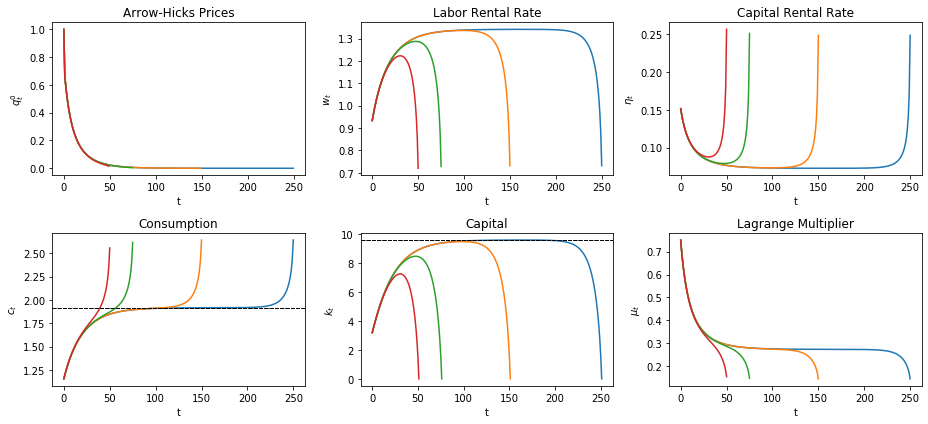#### Varying Curvature¶

Now we see how our results change if we keep $T$ constant, but allow the curvature parameter, $\gamma$ to vary, starting with $K_0$ below the steady state.

We plot the results for $T=150$

In :
γ_list = (1.1, 4, 6, 8)
T = 150

fix, axes = plt.subplots(2, 3, figsize=(13, 6))

for γ in γ_list:
c = np.zeros(T+1)
k = np.zeros(T+2)
c = 0.3
k = k_ss / 3
c, k, μ = bisection_method(c, k, γ, δ, β, α, A)

q = q_func(β, c, γ)
w = w_func(β, k, α)[:-1]
η = η_func(A, k, α)[:-1]
plots = [q, w, η, c, k, μ]

for ax, plot, title, y in zip(axes.flatten(), plots, titles, ylabels):
ax.plot(plot, label=f'$\gamma = {γ}$')
ax.set(title=title, ylabel=y, xlabel='t')
if title is 'Capital':
ax.axhline(k_ss, lw=1, ls='--', c='k')
if title is 'Consumption':
ax.axhline(c_ss, lw=1, ls='--', c='k')

axes[0, 0].legend()
plt.tight_layout()
plt.show()

Converged successfully on iteration 44
Converged successfully on iteration 37
Converged successfully on iteration 37
Converged successfully on iteration 37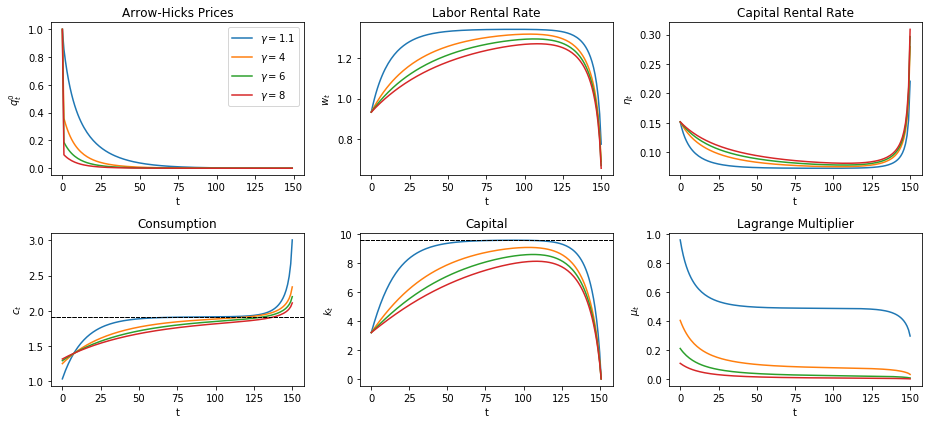Adjusting $\gamma$ means adjusting how much individuals prefer to smooth consumption.

Higher $\gamma$ means individuals prefer to smooth more resulting in slower adjustments to the steady state allocations.

Vice-versa for lower $\gamma$.

### Yield Curves and Hicks-Arrow Prices Again¶

Now, we compute Hicks-Arrow prices again, but also calculate the implied yields to maturity.

This will let us plot a yield curve.

The key formulas are:

The yield to maturity

$$r_{t_0,t}= -\frac{\log q^{t_0}_t}{t - t_0}$$

A generic Hicks-Arrow price for any base-year $t_0\leq t$

$$q^{t_0}_t = \beta^{t-t_0} \frac{u'(c_t)}{u'(c_{t_0})}= \beta^{t-t_0} \frac{c_t^{-\gamma}}{c_{t_0}^{-\gamma}}$$

We redefine our function for $q$ to allow arbitrary base years, and define a new function for $r$, then plot both.

First, we plot when $t_0=0$ as before, for different values of $T$, with $K_0$ below the steady state

In :
@njit
def q_func(t_0, β, c, γ):
# Here we choose numeraire to be u'(c_0) -- this is q^(t_0)_t
T = len(c)
q = np.zeros(T+1-t_0)
q = 1
for t in range(t_0+1, T):
q[t-t_0] = β**(t - t_0) * u_prime(c[t], γ) / u_prime(c[t_0], γ)
return q

@njit
def r_func(t_0, β, c, γ):
'''Yield to maturity'''
T = len(c) - 1
r = np.zeros(T+1-t_0)
for t in range(t_0+1, T+1):
r[t-t_0]= -np.log(q_func(t_0, β, c, γ)[t-t_0]) / (t - t_0)
return r

t_0 = 0
T_list = [150, 75, 50]
γ = 2
titles = ['Hicks-Arrow Prices', 'Yields']
ylabels = ['$q_t^0$', '$r_t^0$']

fig, axes = plt.subplots(1, 2, figsize=(10, 5))

for T in T_list:
c = np.zeros(T+1)
k = np.zeros(T+2)
c = 0.3
k = k_ss / 3
c, k, μ = bisection_method(c, k, γ, δ, β, α, A)
q = q_func(t_0, β, c, γ)
r = r_func(t_0, β, c, γ)

for ax, plot, title, y in zip(axes, (q, r), titles, ylabels):
ax.plot(plot)
ax.set(title=title, ylabel=y, xlabel='t')

plt.tight_layout()
plt.show()

Converged successfully on iteration 39
Converged successfully on iteration 26
Converged successfully on iteration 25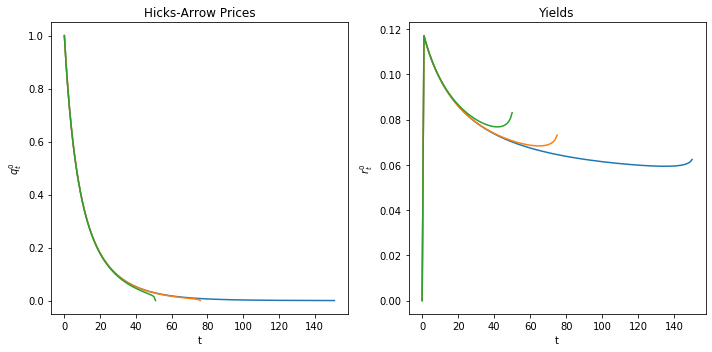Now we plot when $t_0=20$

In :
t_0 = 20

fig, axes = plt.subplots(1, 2, figsize=(10, 5))

for T in T_list:
c = np.zeros(T+1)
k = np.zeros(T+2)
c = 0.3
k = k_ss / 3
c, k, μ = bisection_method(c, k, γ, δ, β, α, A)
q = q_func(t_0, β, c, γ)
r = r_func(t_0, β, c, γ)

for ax, plot, title, y in zip(axes, (q, r), titles, ylabels):
ax.plot(plot)
ax.set(title=title, ylabel=y, xlabel='t')

axes.set_title(f'Yields at $t_0 = {t_0}$')
plt.tight_layout()
plt.show()

Converged successfully on iteration 39
Converged successfully on iteration 26
Converged successfully on iteration 25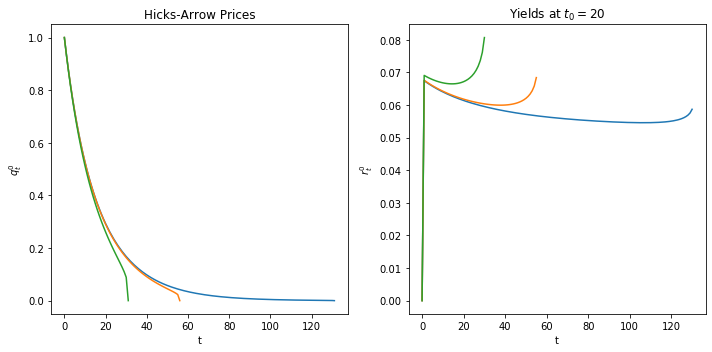We shall have more to say about the term structure of interest rates in a later lecture on the topic.

• Share page